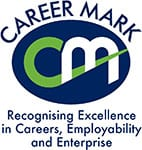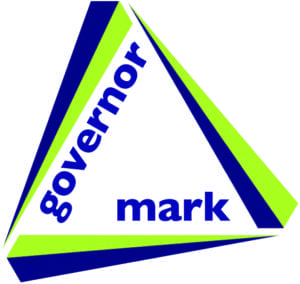# Core Maths

### Why choose this subject?

This qualification is designed to consolidate and build on mathematical understanding at GCSE, as well as further developing students’ mathematical understanding and skills in the application of Mathematics to authentic problems.

The objectives of the course:
• Deepen competence in the selection and use of mathematical techniques used at GCSE and beyond.
• Develop confidence in representing and analysing authentic situations mathematically and applying Mathematics to address related questions and issues.
• Build skills in mathematical thinking, reasoning and communication.

### How will this subject be delivered?

Mathematics is taught through theoretical lessons. Students will develop problem solving and analytical skills as the course develops.

### How is it assessed?

Assessment is by two exams – one 2 hours long and one 1 hour 30 minutes in duration. Both exams will contain questions based on pre-release material that is given two months in advance. There will be real life problems addressed such as Income Tax and National Insurance payments.

Paper 1: Quantitative Methods – financial mathematics, probability and risk, graph analysis, normal distribution.
Paper 2: Critical Analysis – unseen problem solving, Fermi estimations, analysis and evaluation of data, mathematical modelling.

Qualification: • Level 3 (equivalent to AS Level). • One year course.

### Subject specific entry requirements

• A minimum of grade 4 at GCSE Mathematics.

### Progression

This is a new government and employer sponsored course. It will help students progress mathematically and gain the skills they will need to complete university courses and to compete in emerging employer markets. In particular, it is important to students looking to progress to higher education courses with distinct mathematical or statistical elements, such as Product Design, Economics, Business, Geography, Science and Engineering. Core Mathematics will be invaluable for any students aiming for a career in a professional or technical field.

### Additional costs relating to this course

• Scientific calculator and textbook

Level: AS LEVEL –  1 YEAR COURSE

Board: OCR